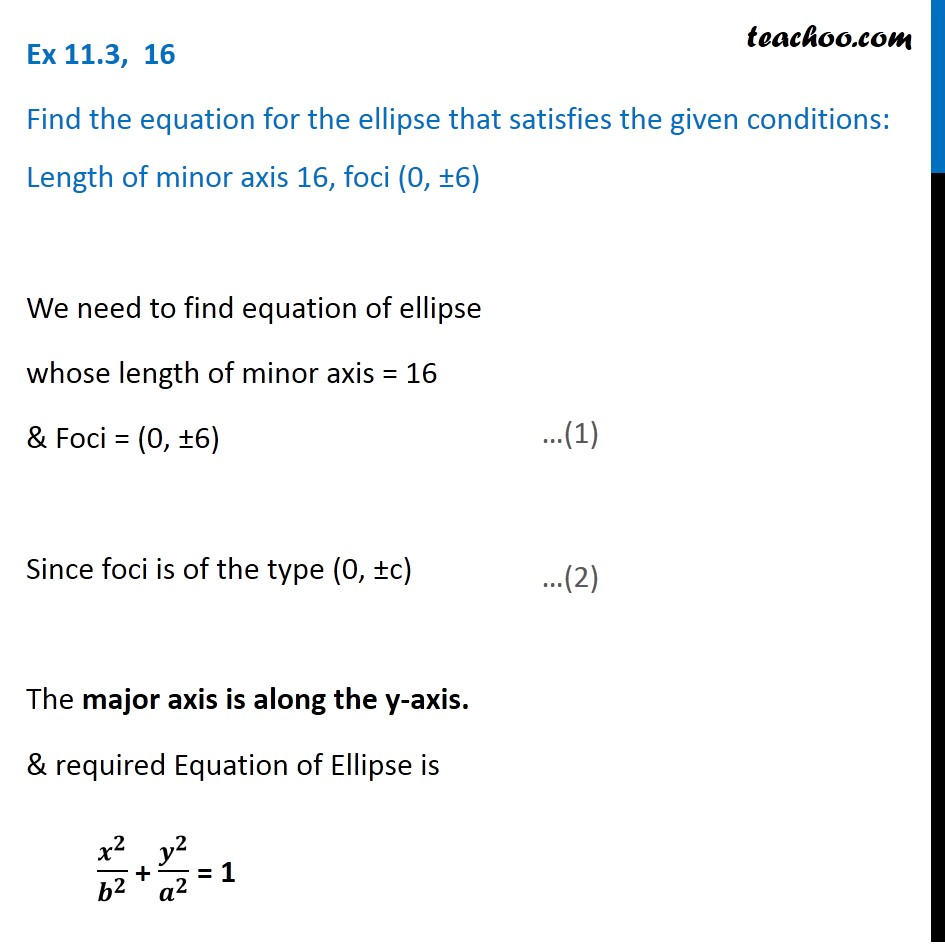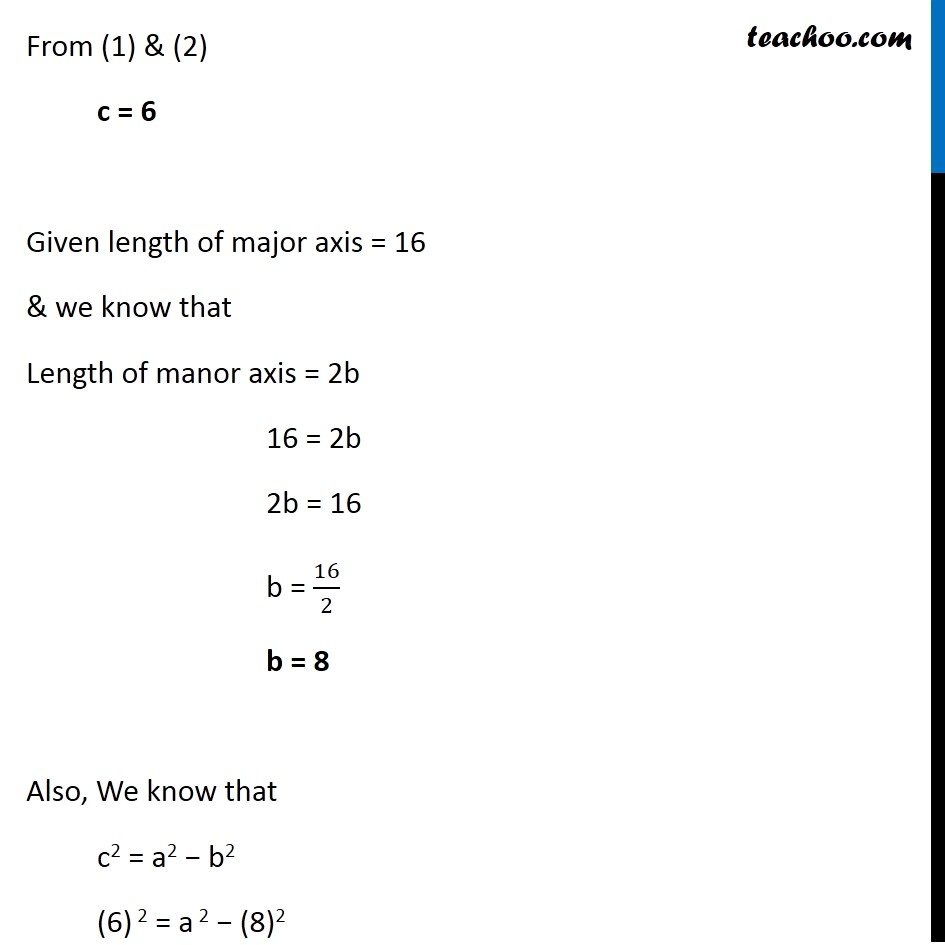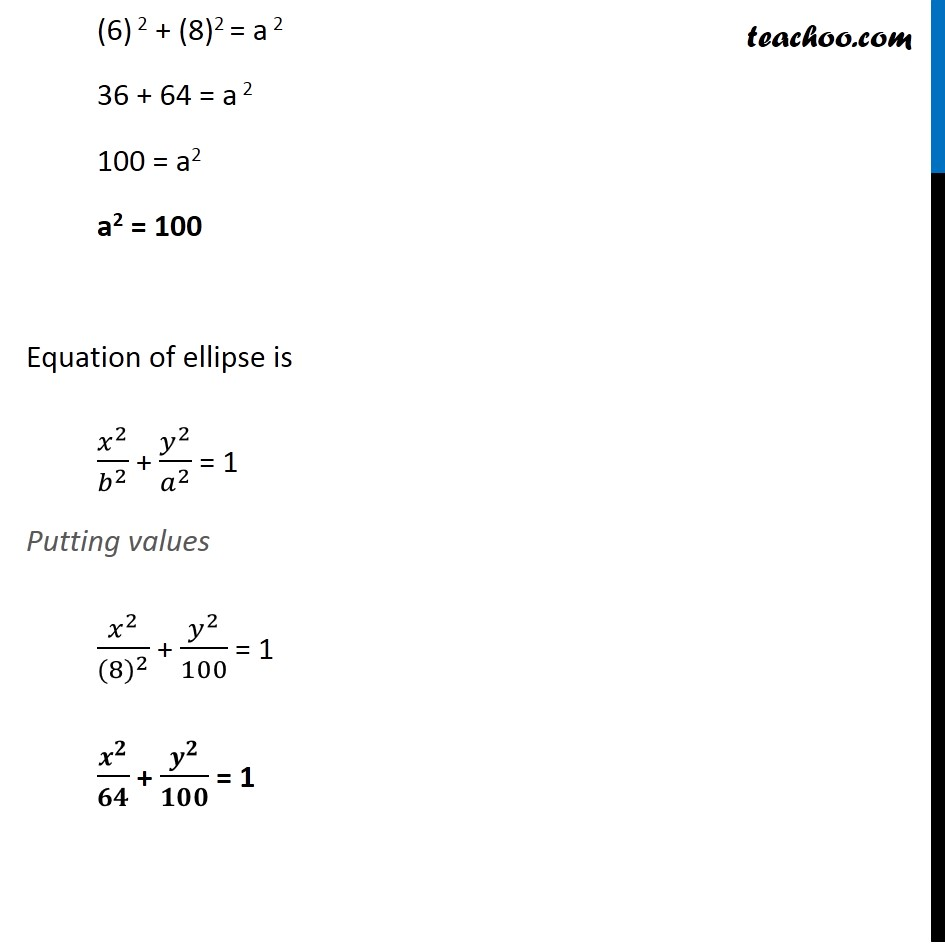Ex 10.3

Chapter 10 Class 11 Conic Sections
Serial order wiseLearn in your speed, with individual attention - Teachoo Maths 1-on-1 Class

### Transcript

Ex 10.3, 16 Find the equation for the ellipse that satisfies the given conditions: Length of minor axis 16, foci (0, ±6) We need to find equation of ellipse whose length of minor axis = 16 & Foci = (0, ±6) Since foci is of the type (0, ±c) The major axis is along the y-axis. & required Equation of Ellipse is 𝒙^𝟐/𝒃^𝟐 + 𝒚^𝟐/𝒂^𝟐 = 1 From (1) & (2) c = 6 Given length of major axis = 16 & we know that Length of manor axis = 2b 16 = 2b 2b = 16 b = 16/2 b = 8 Also, We know that c2 = a2 − b2 (6) 2 = a 2 − (8)2 (6) 2 + (8)2 = a 2 36 + 64 = a 2 100 = a2 a2 = 100 Equation of ellipse is 𝑥^2/𝑏^2 + 𝑦^2/𝑎^2 = 1 Putting values 𝑥^2/〖(8)〗^2 + 𝑦^2/100 = 1 𝒙^𝟐/𝟔𝟒 + 𝒚^𝟐/𝟏𝟎𝟎 = 1